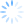Login/Register Math Worksheets, Activities, Games Helpline 099826-99306

# Grade 4 Math Worksheets: Printable worksheet for 4th grade maths students

Continuing our series on math worksheets, we present math worksheets for grade 4. The complexity and skill requirement in grade 4 math increases in each and every topic. In grade 4, curriculum moves to advanced levels of addition, subtraction, multiplication and division. Math students are also exposed to more conceptual and application based topics such as unitary methods and metric system, area and perimeter and fractions.

It would help a great deal if you can integrate Activity, Fun and Real life application of Math while staying true to the curriculum. The focus should be show a sense of achievement, accomplishment and fun at the end of the activity. Below we present 3 sample math worksheets for grade 4. Each worksheet has a major math focus and a minor focus depending upon the grade 4 math syllabus. Read on to discover more.

## 1st worksheet for Grade 4 Math: Remainders in division

"Accountant Owl" math worksheet integrates the story of owl and the birds with division practice.Worksheet focus

• Grade for this worksheet: grade 4 Math
• Major focus of this worksheet: Remainders in division
• Minor focus of this worksheet: Application of remainders

Click here to download more printable math worksheets for grade 4. These worksheets and activities are aligned to NCERT India and NCTM singapore curriculum for grade 4.

## 2nd worksheet for Grade 4 Math: Distances and lengths

With "Coconutty Tail" BrainX team combines the concepts of distances with knowledge of division. The worksheet also has a little "trap" where many students might go wrong. Try it to see if you can spot the trapWorksheet focus

• Grade for this worksheet: Class 4 Maths
• Major focus of this worksheet: Distances and lengths, Division
• Minor focus of this worksheet: Common analytics

As a parent or teacher, your focus should be less on solving math sums and more on understanding. It is a good idea is you bring in the fun element, say some math trick that appears like a magic or may be some activity that you can make them do at home involving colours, glue etc. The idea is that math becomes more "touchy" and "feely" rather than a complex subject.

## 3rd worksheet for Grade 4 Math: BODMAS

BODMAS in class 4 is a very important skill to master for student to do multi step arithmetic operations. The students have to understand the sequence of applying arithmetic operators. So BODMAS stands for Bracket, Of, Divide, Multiply, Add and Subtract. These operations must be carried in the sequence of BODMAS. "Earth Calling Apollo" provides a  captivating situation while the student practices BODMASWorksheet focus

• Grade for this worksheet: Class 4 Maths
• Major focus of this worksheet: BODMAS

When you address conceptual math topics such as BODMAS, it would help if you bring out a real life application. For example, did you know that the computing power of Apollo-1 rockets was even less than pocket calculators of this date. So they could not code or store a lot of information and hence they made simple rules. NOTE - BODMAS came much before Apollo-1 but this background might help to explain the story.

Hope this was of some help. You can find more free math worksheets sorted by grade on our website. Try them and have fun because Math is to enjoyed not feared.